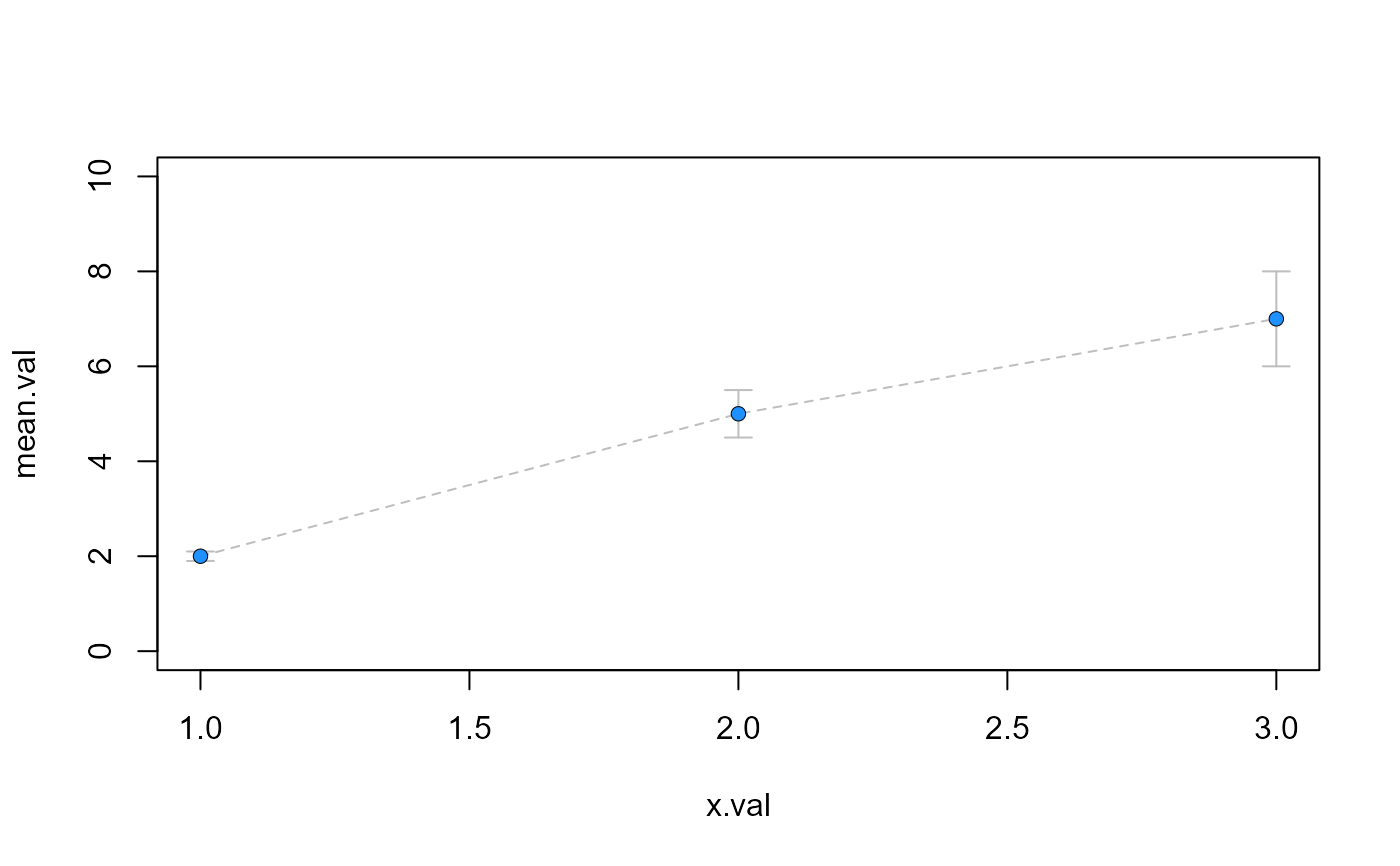Point with error bars

pt_line_error(
x,
y,
SE,
ln.lty,
ln.col,
ln.lwd,
pch,
bg,
cex = 1,
pt.lwd = 1,
pt.lty = 1,
er.lwd = 1,
pt.col = "black",
length = 0.07
)

## Arguments

x x-value y-value (or mean) standard error line type color of lines width of lines plotting ‘character’, i.e., symbol to use. see points() background (fill) color for the open plot symbols given by pch = 21:25. see points() size of point line width of points line type for points error bar line width color of point length of the edges of the error bar head (in inches).

## Value

draws lines in the form of error bars

## Note

see arrows(), errorbars() and pt_error() functions

## Examples

x.val=c(1,2,3)
mean.val=c(2,5,7)
se.val=c(0.1,0.5,1)
plot(mean.val~x.val,ylim=c(0,10),type="n")pt_line_error(x.val,mean.val,se.val,2,"grey",1,21,"dodgerblue1")